﻿ 一类非局部算子相关的重排优化问题 A Rearrangement Optimization Problem Involving a Nonlocal Operator

Pure Mathematics
Vol. 09  No. 06 ( 2019 ), Article ID: 31737 , 7 pages
10.12677/PM.2019.96099

A Rearrangement Optimization Problem Involving a Nonlocal Operator

Chong Qiu1, Yuying Zhou2

1School of Mathematics and Physics, TaiZhou University, Taizhou Jiangsu

2School of Mathematical Sciences, Soochow University, Suzhou Jiangsu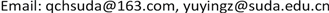Received: Jul. 21st, 2019; accepted: Jul. 31st, 2019; published: Aug. 16th, 2019ABSTRACT

In this paper, we study a rearrangement optimization problem involving a nonlocal operator, i.e., fractional Laplacian. Firstly, we use the property of the first eigenvalue to prove that the nonlinear equation with a perturbation term involving the fractional Laplacian has a unique solution. Then, we introduce an optimization problem which takes the ground state energy functional as the objective function. We show that under suitable assumptions such an optimization problem is solvable.

Keywords:Nonlocal Operator, Rearrangement Optimization, Perturbation Term

1泰州学院数理学院，江苏 泰州

2苏州大学数学科学学院，江苏 苏州1. 引言

$\Omega \subset {R}^{N}$ 为一个有界光滑区域，所谓 $\Omega$ 上可测函数f生成的重排函数空间 $R\left(f\right)$ 是指由所有满足条件：

$meas\left(\left\{x\in \Omega :g\left(x\right)\ge a\right\}\right)=meas\left(\left\{x\in \Omega :f\left(x\right)\ge a\right\}\right),\forall a\in R$

$\left\{\begin{array}{l}-{L}_{\theta }^{s}u=f\left(x\right)+h\left(x,u\right),x\in \Omega \\ u=0,x\in {R}^{N}\\Omega \end{array}$ (1.1)

${L}_{\theta }^{s}u\left(x\right)={\int }_{{R}^{N}}\frac{u\left(x+y\right)+u\left(x-y\right)-2u\left(x\right)}{{|y|}^{N+2s}}\theta \left(y\right)\text{d}y,x\in {R}^{N}$ ,

$\left({P}_{\lambda ,h,f}\right)$ $\left\{\begin{array}{l}{\left(-\Delta \right)}^{s}u=f\left(x\right)+\lambda h\left(x\right)u,x\in \Omega \\ u=0,x\in {R}^{N}\\Omega \end{array}$

$I:{H}^{s}\left(\Omega \right)\to R$ 为方程 $\left({P}_{\lambda ,h,f}\right)$ 的能量泛函，即

$I\left(u\right)=\frac{1}{2}{\int }_{{R}^{N}}{\int }_{{R}^{N}}\frac{{\left(u\left(x\right)-u\left(y\right)\right)}^{2}}{{|x-y|}^{N+2s}}\text{d}x\text{d}y-\frac{\lambda }{2}{\int }_{\Omega }h{u}^{2}\text{d}x-{\int }_{\Omega }fu\text{d}x$ (1.2)

(Opt) $I\left({u}_{\stackrel{^}{h},\stackrel{^}{f}}\right)={\mathrm{inf}}_{h\in R\left({h}_{0}\right),f\in R\left({f}_{0}\right)}I\left({u}_{h,f}\right)$ ?

2. 预备知识

${H}^{s}\left(\Omega \right)=\left\{u\in {L}^{2}\left({R}^{N}\right):u\equiv 0,x\in {R}^{N}\\Omega ,{\int }_{{R}^{N}}{\int }_{{R}^{N}}\frac{{\left(u\left(x\right)-u\left(y\right)\right)}^{2}}{{|x-y|}^{N+2s}}\text{d}x\text{d}y<\infty \right\}$ ,

$〈u,v〉={\int }_{{R}^{N}}{\int }_{{R}^{N}}\frac{\left(u\left(x\right)-u\left(y\right)\right)\left(v\left(x\right)-v\left(y\right)\right)}{{|x-y|}^{N+2s}}\text{d}x\text{d}y,\forall u,v\in {H}^{s}\left(\Omega \right)$ .

$u\in {H}^{s}\left(\Omega \right)$ ，则u的范数为

$‖u‖={\left({\int }_{{R}^{N}}{\int }_{{R}^{N}}\frac{{\left(u\left(x\right)-u\left(y\right)\right)}^{2}}{{|x-y|}^{N+2s}}\text{d}x\text{d}y\right)}^{\frac{1}{2}}$ .

${\int }_{{R}^{N}}{\int }_{{R}^{N}}\frac{\left(u\left(x\right)-u\left(y\right)\right)\left(v\left(x\right)-v\left(y\right)\right)}{{|x-y|}^{N+2s}}\text{d}x\text{d}y-{\int }_{\Omega }\left(\lambda huv+fv\right)\text{d}x=0,\forall v\in {H}^{s}\left(\Omega \right)$ .

${I}^{\prime }\left(u\right)v={\int }_{{R}^{N}}{\int }_{{R}^{N}}\frac{\left(u\left(x\right)-u\left(y\right)\right)\left(v\left(x\right)-v\left(y\right)\right)}{{|x-y|}^{N+2s}}\text{d}x\text{d}y-{\int }_{\Omega }\left(\lambda huv+fv\right)\text{d}x,\forall v\in {H}^{s}\left(\Omega \right)$ .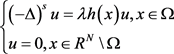(2.1)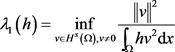(2.2)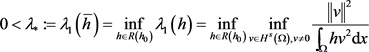. (2.3)

3. 方程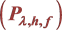解的存在唯一性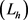的第一特征值，则方程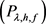存在唯一解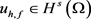，且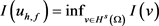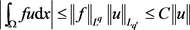,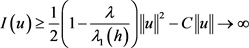, 若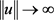,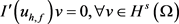.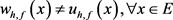. (2.4)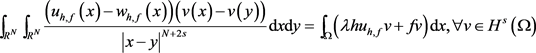,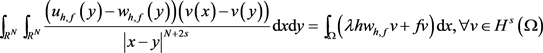.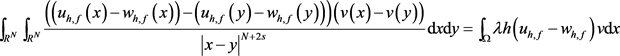..

4. 重排优化问题(Opt)的可解性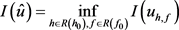,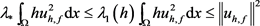.. (4.1)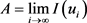,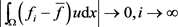.. (4.2)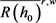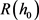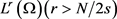中的弱闭包。因为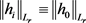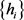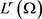中有界，所以存在子列(不妨仍记为)弱收敛于某个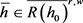。通过类似地论证可以得到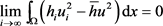. (4.3)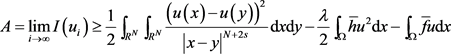(4.4)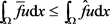.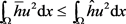.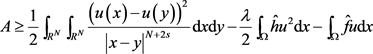. (4.5). (4.6)

A Rearrangement Optimization Problem Involving a Nonlocal Operator[J]. 理论数学, 2019, 09(06): 755-761. https://doi.org/10.12677/PM.2019.96099

1. 1. Burton, G.R. (1987) Rearrangements of Functions, Maximization of Convex Functionals and Vortex Rings. Mathematische Annalen, 276, 225-253.
https://doi.org/10.1007/BF01450739

2. 2. Burton, G.R. (1989) Variational Problems on Classes of Rearrange-ments and Multiple Configurations for Steady Vortices. Annales de l’Institut Henri Poincaré, 6, 295-319.
https://doi.org/10.1016/S0294-1449(16)30320-1

3. 3. Cuccu, F., Emamizadeh, B. and Porru, G. (2006) Nonlinear Elastic Membrane Involving the p-Laplacian Operator. Electronic Journal of Differential Equations, 49, 1-10.

4. 4. Cuccu, F., Emamizadeh, B. and Porru, G. (2009) Optimization of the First Eigenvalue in Problems Involving the p-Laplacian. Proceedings of the AMS, 137, 1677-1687.
https://doi.org/10.7153/dea-01-12

5. 5. Cuccu, F., Porru, G. and Sakaguchi, S. (2011) Optimization Problems on General Classes of Rearrangements. Nonlinear Analysis, 74, 5554-5565.
https://doi.org/10.1016/j.na.2011.05.039

6. 6. Emamizadeh, B. and Zivari-Rezapour, M. (2007) Rearrangement Optimization for Some Elliptic Equations. Journal of Optimization Theory and Applications, 135, 367-379.
https://doi.org/10.1007/s10957-007-9269-y

7. 7. Emamizadeh, B. and Prajapat, J.V. (2009) Symmetry in Rearrangemet Opti-mization Problems. Electronic Journal of Differential Equations, 149, 1-10.

8. 8. Marras, M. (2010) Optimization in Problems In-volving the p-Laplacian. Electronic Journal of Differential Equations, 2, 1-10.

9. 9. Qiu, C., Huang, Y.S. and Zhou, Y.Y. (2015) A Class of Rearrangement Optimization Problems Involving the p-Laplacian. Nonlinear Analysis, 112, 30-42.
https://doi.org/10.1016/j.na.2014.09.008

10. 10. Qiu, C., Huang, Y.S. and Zhou, Y.Y. (2016) Optimization Problems Involving the Fractional Laplacian. Electronic Journal of Differential Equations, 2016, 1-15.

11. 11. Pezzo, L.D., Bonder, J.F. and Rios, L.L. (2018) An Optimization Problem for the First Eigenvalue of the p-Fractional Laplacian. Mathematische Nachrichten, 291, 632-651.

12. 12. Dalibard, A.L. and Gerard-Varet, D. (2013) On Shape Optimization Problems Involving the Fractional Laplacian. ESAIM: Control, Optimisation and Calculus of Variations, 19, 976-1013.
https://doi.org/10.1051/cocv/2012041

13. 13. Servadei, R. and Valdinoci, E. (2012) Mountain Pass Solutions for Non-Local Elliptic Operators. Journal of Mathematical Analysis and Ap-plications, 389, 887-898.
https://doi.org/10.1016/j.jmaa.2011.12.032

14. 14. 邱崇. 几类与非线性椭圆型方程相关的重排优化问题研究[D]: [博士学位论文]. 苏州: 苏州大学数学科学学院, 2015.